Ex 2.5

Chapter 2 Class 7 Fractions and Decimals
Serial order wise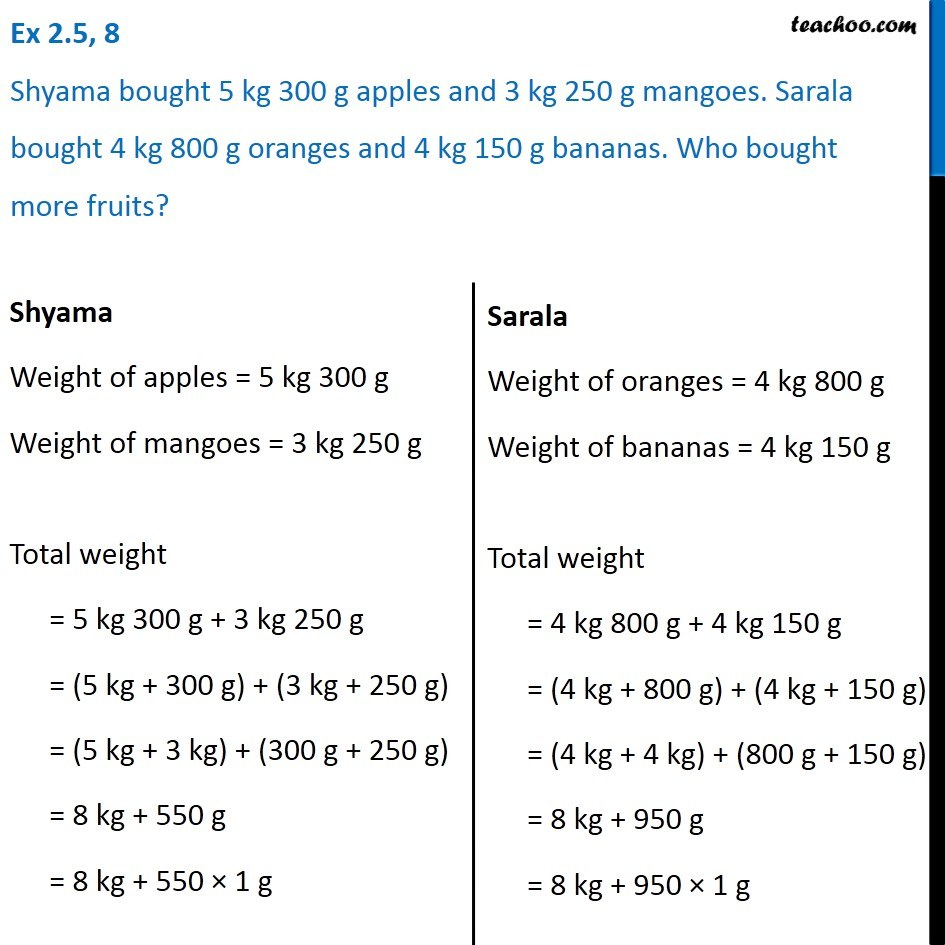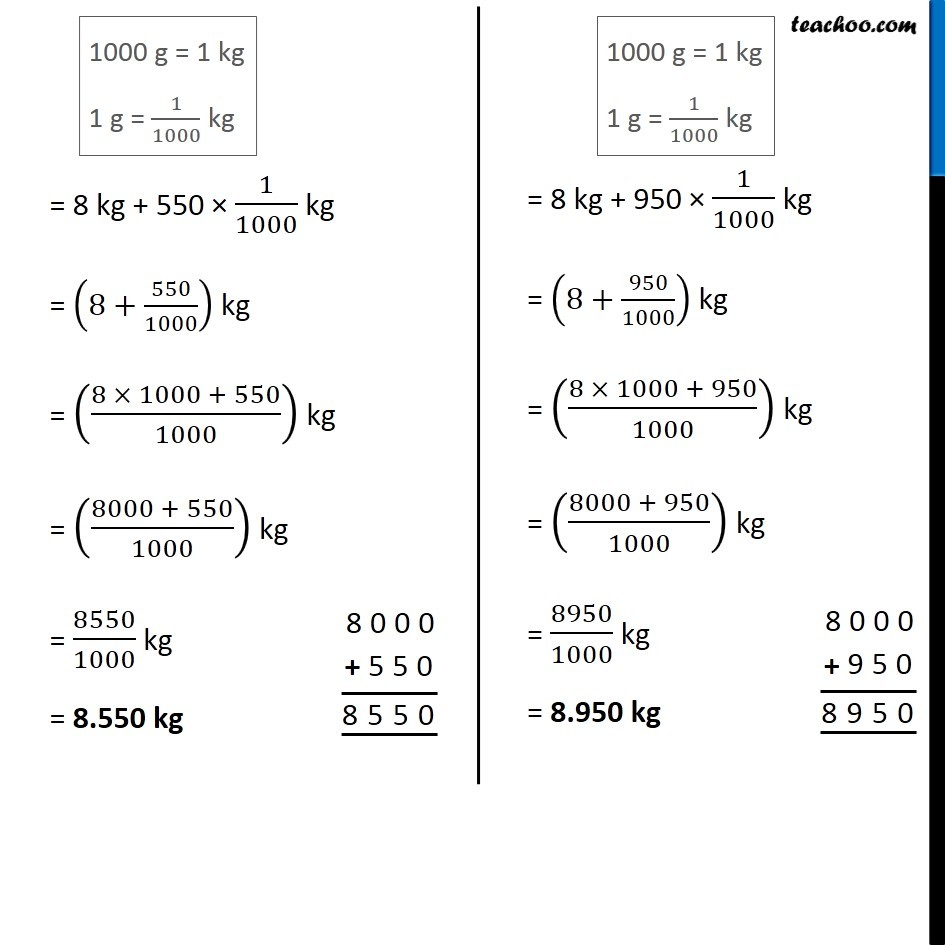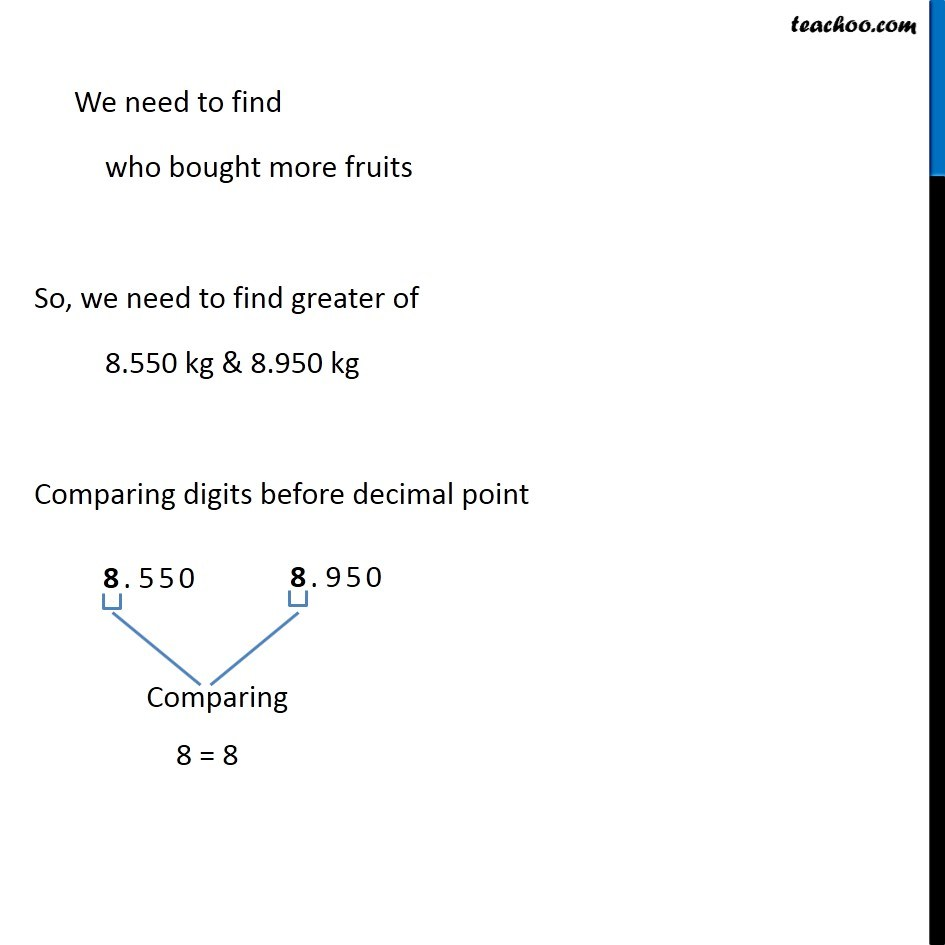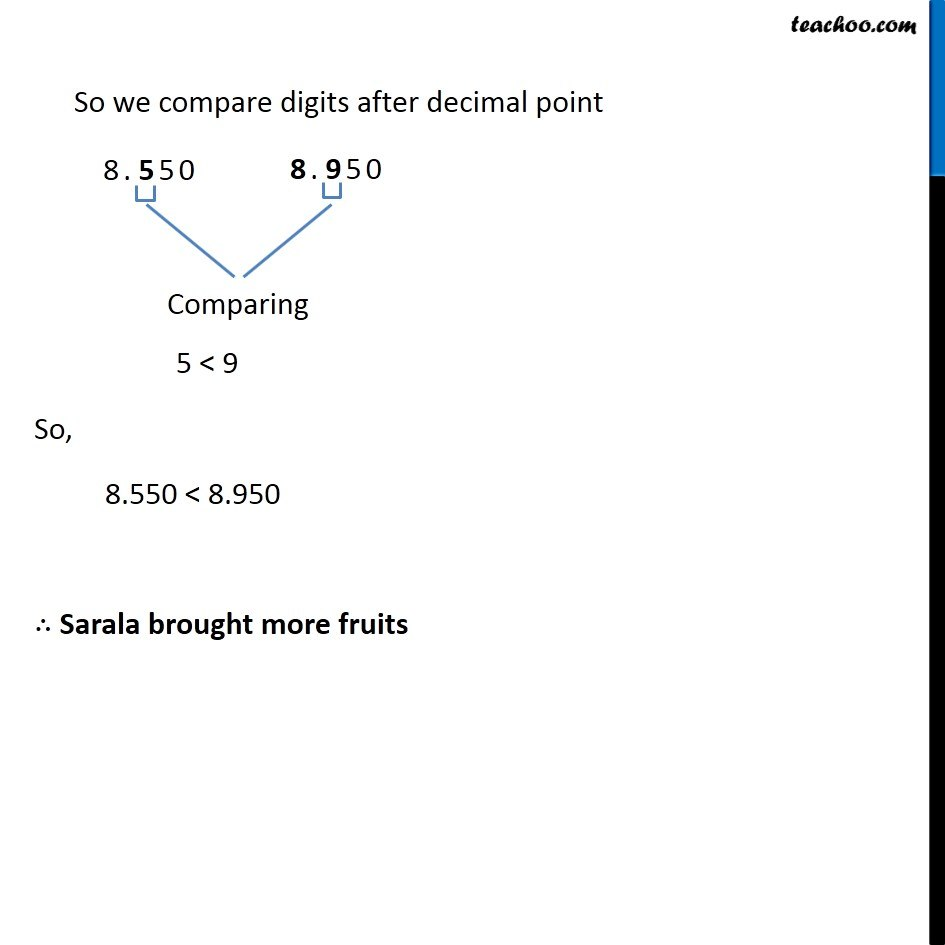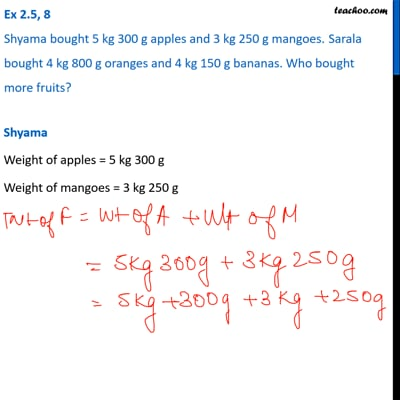This video is only available for Teachoo black users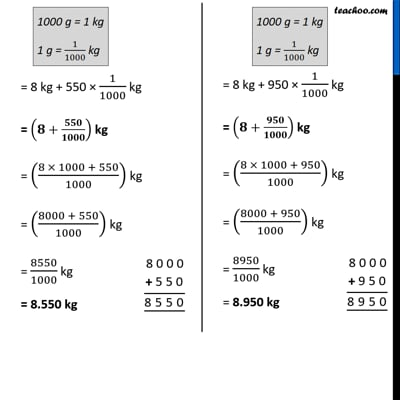This video is only available for Teachoo black users

Get live Maths 1-on-1 Classs - Class 6 to 12

### Transcript

Ex 2.5, 8 Shyama bought 5 kg 300 g apples and 3 kg 250 g mangoes. Sarala bought 4 kg 800 g oranges and 4 kg 150 g bananas. Who bought more fruits?Shyama Weight of apples = 5 kg 300 g Weight of mangoes = 3 kg 250 g Total weight = 5 kg 300 g + 3 kg 250 g = (5 kg + 300 g) + (3 kg + 250 g) = (5 kg + 3 kg) + (300 g + 250 g) = 8 kg + 550 g = 8 kg + 550 × 1 g Sarala Weight of oranges = 4 kg 800 g Weight of bananas = 4 kg 150 g Total weight = 4 kg 800 g + 4 kg 150 g = (4 kg + 800 g) + (4 kg + 150 g) = (4 kg + 4 kg) + (800 g + 150 g) = 8 kg + 950 g = 8 kg + 950 × 1 g 1000 g = 1 kg 1 g = 1/1000 kg = 8 kg + 550 × 1/1000 kg = (8+550/1000) kg = ((8 × 1000 + 550)/1000) kg = ((8000 + 550)/1000) kg = 8550/1000 kg = 8.550 kg 1000 g = 1 kg 1 g = 1/1000 kg = 8 kg + 950 × 1/1000 kg = (8+950/1000) kg = ((8 × 1000 + 950)/1000) kg = ((8000 + 950)/1000) kg = 8950/1000 kg = 8.950 kg We need to find who bought more fruits So, we need to find greater of 8.550 kg & 8.950 kg Comparing digits before decimal point 8 = 8 So we compare digits after decimal point 5 < 9 So, 8.550 < 8.950 ∴ Sarala brought more fruits